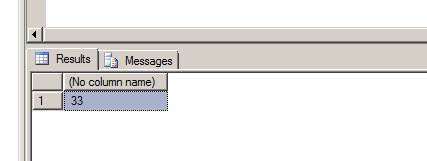# How to Calculate Age in Ms-Sql server with SQL Example

Category > MYSQL || Published on : Monday, August 25, 2014 || Views: 10537 || Calcluate age using SQL SQL query to get age Sql Tip

Introduction:-

Here Pawan Kumar has explained, How to Calculate Age in Ms-Sql server with SQL Example

In some condtions, We have to calculate the age of the employee so  for that we have to calculate the age into the C#  or also we can calculate age in Ms-Sql server. For this we can create a function in Ms-Sql server and when ever we find the need we can use that function.

Write the following SQL Query into SQL Server Management Studio. In this condition, I'm using the Ms-Sql server 2008 Edition.

This simple SQL will help you to calcuate you age using Ms-Sql server

```DECLARE @BirthDate DATETIME
DECLARE @CurrentDate DATETIME

SELECT @CurrentDate = getdate(), @BirthDate = '08/21/1981' --Add your date of birth here

SELECT DATEDIFF(YY, @BirthDate, @CurrentDate) -
CASE
WHEN( (MONTH(@BirthDate)*100 + DAY(@BirthDate)) > (MONTH(@CurrentDate)*100 + DAY(@CurrentDate)) )
THEN 1 ELSE 0 END```

Output will be like thisso guys, we have learned in this tutorial, How to Calculate Age in Ms-Sql server with SQL Example